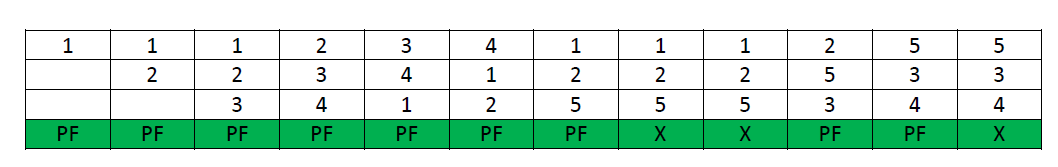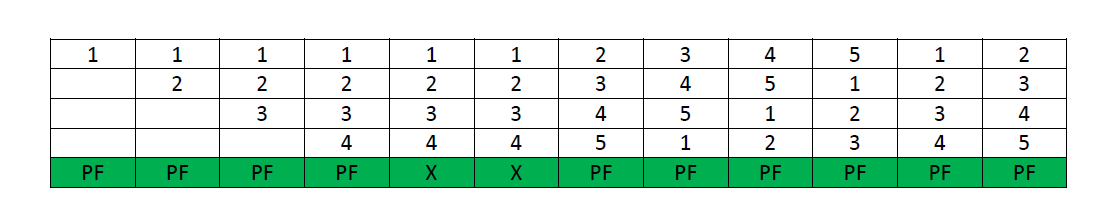# GATE2009-9, ISRO2016-52

4k views

In which one of the following page replacement policies, Belady's anomaly may occur?

1. FIFO
2. Optimal
3. LRU
4. MRU

edited
0

Consider the following reference string:

1, 2, 3, 4, 1, 2, 5, 1, 2, 3, 4, 5

Case-1: If the system has 3 frames, the given reference string on using FIFO page replacement algorithm yields a total of 9 page faults. The diagram below illustrates the pattern of the page faults occurring in the example.Case-2: If the system has 4 frames, the given reference string on using FIFO page replacement algorithm yields a total of 10 page faults. The diagram below illustrates the pattern of the page faults occurring in the example.It can be seen from the above example that on increasing the number of frames while using the FIFO page replacement algorithm, the number of page faults increased from 9 to 10.

edited by

In computer storageBélády's anomaly is the phenomenon in which increasing the number of page frames results in an increase in the number of page faults for certain memory access patterns. This phenomenon is commonly experienced when using the First in First Out (FIFOpage replacement algorithm.

So, ans is (A)FIFO

How/Why?
Page Replacement algorithms suffer from Belady’s anamoly if :

• They do not follow the stack based algorithm.

Reference :-

Since MRU, LRU & Optimal Replacement Algo follow Stack Algo, hence they are not affected by Belady's Anamoly.

edited
Option:( A) FIFO

Belady's anomaly is the problem which occur when we increase the frame size and still the page fault increases

## Related questions

1
22.1k views
Frames of $\text{1000 bits}$ are sent over a $10^6$ $\text{bps}$ duplex link between two hosts. The propagation time is $\text{25 ms}$. Frames are to be transmitted into this link to maximally pack them in transit (within the link). What is the minimum number of bits ... numbers distinctly? Assume that no time gap needs to be given between transmission of two frames. $I=2$ $I=3$ $I=4$ $I=5$
$S \to aSa \mid bSb\mid a\mid b$ The language generated by the above grammar over the alphabet $\{a,b\}$ is the set of: all palindromes all odd length palindromes strings that begin and end with the same symbol all even length palindromes
A hard disk has $63$ sectors per track, $10$ platters each with $2$ recording surfaces and $1000$ cylinders. The address of a sector is given as a triple $\langle c, h, s \rangle$, where $c$ is the cylinder number, $h$ is the surface number and $s$ is the sector number. Thus, the ... is $\langle 0, 15, 31 \rangle$ $\langle 0, 16, 30 \rangle$ $\langle 0, 16, 31 \rangle$ $\langle 0, 17, 31 \rangle$